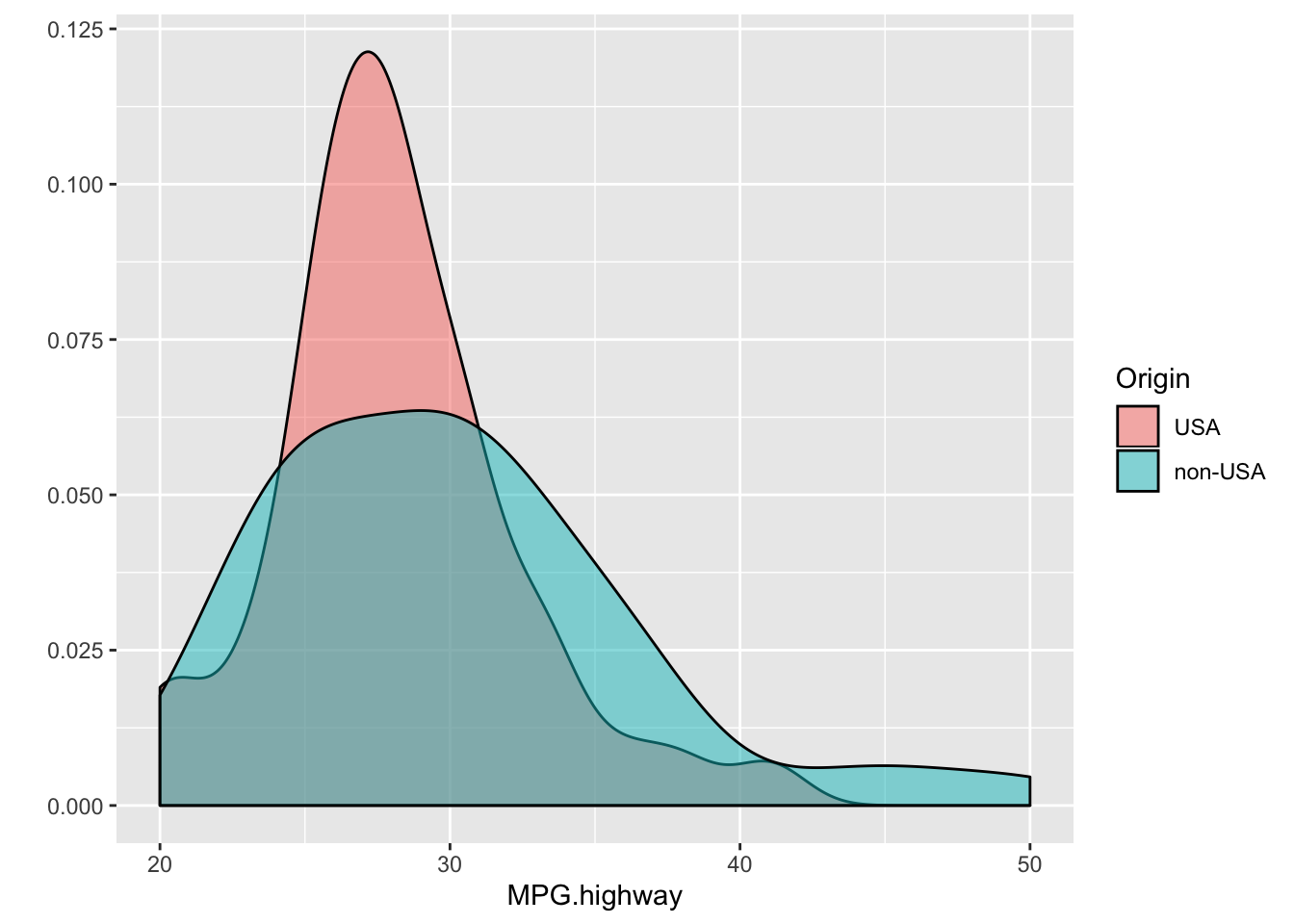### Learning objectives

In today’s Lab you will gain practice with the following concepts from today’s class:

• Using the t.test and wilcox.test commands to run 2-sample t-tests
• Interpreting the results of statistical significance tests
• Using qqnorm and qqline to construct normal quantile-quantile plots, and using them to assess whether data appear to be normally distributed
• Using fisher.test on 2x2 tables and interpreting the results

library(tidyverse)
## ── Attaching packages ──────────────────────────────── tidyverse 1.2.1 ──
## ✔ ggplot2 3.2.1     ✔ purrr   0.3.3
## ✔ tibble  2.1.3     ✔ dplyr   0.8.3
## ✔ tidyr   1.0.0     ✔ stringr 1.4.0
## ✔ readr   1.3.1     ✔ forcats 0.4.0
## ── Conflicts ─────────────────────────────────── tidyverse_conflicts() ──
## ✖ dplyr::lag()    masks stats::lag()
Cars93 <- as_tibble(MASS::Cars93)

### Testing means between two groups

Here is a command that generates density plots of MPG.highway from the Cars93 data. Separate densities are constructed for US and non-US vehicles.

qplot(data = Cars93, x = MPG.highway,
fill = Origin, geom = "density", alpha = I(0.5))(a) Using the Cars93 data and the t.test() function, run a t-test to see if average MPG.highway is different between US and non-US vehicles. Interpret the results

Try doing this both using the formula style input and the x, y style input.

# Edit me

(b) What is the confidence interval for the difference? Interpret this confidence interval.

# Edit me

(c) Repeat part (a) using the wilcox.test() function.

# Edit me

(d) Are your results for (a) and (c) very different?

### Is the data normal?

(a) Modify the density plot code provided in problem 1 to produce a plot with better axis labels. Also add a title.

# Edit me

(b) Does the data look to be normally distributed? If not, describe why.

(c) Construct qqplots of MPG.highway, one plot for each Origin category. Overlay a line on each plot as illustrated in lecture.

# Edit me

(d) Does the data look to be normally distributed? If not, describe why.

### Testing 2 x 2 tables

Doll and Hill’s 1950 article studying the association between smoking and lung cancer contains one of the most important 2 x 2 tables in history.

Here’s their data:

smoking <- as.table(rbind(c(688, 650), c(21, 59)))
dimnames(smoking) <- list(has.smoked = c("yes", "no"),
lung.cancer = c("yes","no"))
smoking
##           lung.cancer
## has.smoked yes  no
##        yes 688 650
##        no   21  59

(a) Use fisher.test() to test if there’s an association between smoking and lung cancer.

# Edit me

(b) What is the odds ratio? Interpret this quantity.

# Edit me

(c) Are your findings statistically significant?

# Edit me

(d) Write an inline code chunk similar to the one you saw in class where you interpret the results of this hypothesis test.